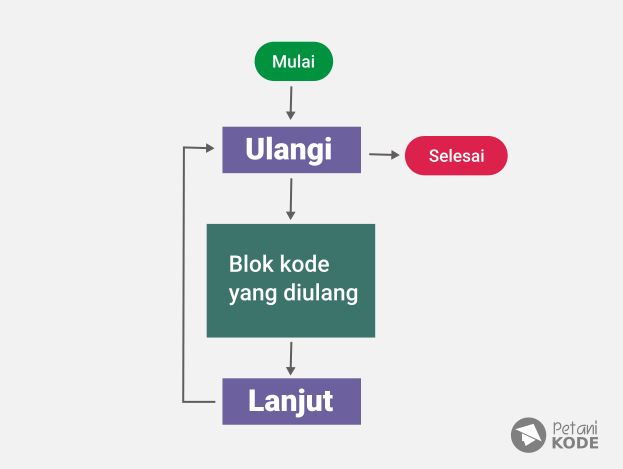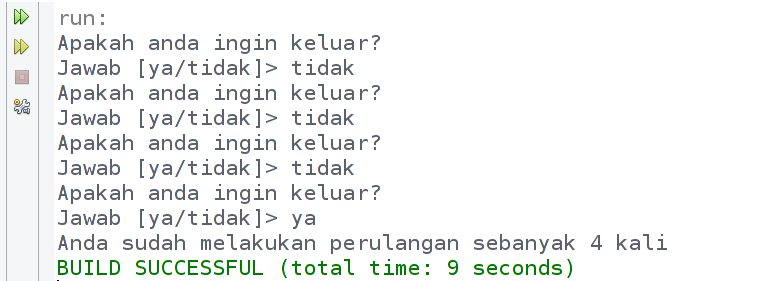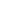| lesson material | material summary | questions and answers | definitions | types and examples | other information | materi pelajaran | ringkasan materi | pertanyaan dan jawaban | definisi | jenis-jenis dan contoh-contoh | informasi lainnya |

# Learning Java: Understanding 2 Types of Repetitions in JavaWhat will we do if we want to have the computer work on repeated commands?
Suppose we want to have a computer display `Petani Kode`5x of text .
Then we can tell it like this:
``````System.out.println("Petani Kode");
System.out.println("Petani Kode");
System.out.println("Petani Kode");
System.out.println("Petani Kode");
System.out.println("Petani Kode");
``````
But ... what about 1000x, will we be able to type that much code?
Of course not.
Therefore, we must use repetition.Examples of repetitions:
``````for (int hitungan = 0; hitungan <= 1000; hitungan++) {
System.out.println("Petani Kode");
}
``````
Before going into deeper discussion, there are things you must know first.
Repetition in programming is divided into two types:
1. Counted loop : Repeat the number of repetitions counted or certain.
2. Uncounted loop : Repetition of which the number of repetitions is uncountable or uncertain.Counted loops consist of repetitions of For and For each . Whereas Uncounted loops consist of repetitions of While and Do / While

## 1. Counted Loop

As explained, this iteration has a certain number of repetitions.
This loop consists of repetition of For and For each .
Let's discuss them one by one…

### Repeat For

The format of repetition writing For Java is as follows:
``````for( int hitungan = 0; hitungan <= 10; hitungan++ ){
// blok kode yang akan diulang
}
``````
Explanation:
• `hitungan`task variable to store repetition counts.
• `hitungan <= 10`meaning that as long as the count value is smaller or equal to 10, then repetitions will continue to be made. In other words, this adventure will repeat 10 times.
• `hitungan++`its function is to add one ( `+1`) value to the count of each repetition.
• The For code block starts with the sign ' `{`' and ends with ' `}`'.
Let's try it in the example program ...

#### Examples of Repetition Programs For

Please create a new class named `Bintang`, then follow the following code:
``````class Bintang{
public static void main(String[] args){

for(int i=0; i <= 5; i++){
System.out.println("*****");
}

}
}
``````
Output results:
``````*****
*****
*****
*****
*****
*****
``````
Want more?
Now try creating a program to display values ​​from 1 to 10 using looping.
``````class CetakAngka{

public static void main(String[] argumen){

for(int i=0; i <= 10; i++){
System.out.print( i + " " );
}

}

}
``````
Output results:
``````0 1 2 3 4 5 6 7 8 9 10
``````
Let's do a little experiment.
Create a program that displays only odd numbers.
``````class CetakBilanganGanjil{

public static void main(String[] argumen){
for(int i = 1; i <= 20; i += 2){
System.out.print( i + " ");
}
}

}
``````
Output results:
``````1 3 5 7 9 11 13 15 17 19
``````
Note: there we use `i += 2`, not `i++`.
What does it mean?
That is, the value `i`will be added by two ( `+2`) in each repetition.

### Repeat For Each

This loop is actually used to display the contents of an array .
What is an array ?
In short, arrays are variables that hold more than one value and have an index.
Continue ...
Repetition For Each in Java, also done with the keyword For .
Examples like this:
``````for ( int item : dataArray ) {
// blok kode yang diulang
}
``````
Explanation:
• variable `item`will store the value of the array
• We can read like this: "For each `item`inside `dataArray`, then repeat"

#### Example Program For Each

Create a new class named `PerulanganForeach`, then follow the following code.
``````public class PerulanganForeach {
public static void main(String[] args) {

// membuat array
int angka[] = {3,1,42,24,12};

// menggunakan perulangan For each untuk menampilkan angka
for( int x : angka ){
System.out.print(x + " ");
}

}
}
``````
The output results:
``````3 1 42 24 12
``````

## Uncounted Loop

As explained earlier, this iteration is not clear the number of repetitions.
But, it does not rule out the possibility, the number of repetitions can be determined.
An uncounted loop consists of repetitions of While and Do / While .Let's discuss them one by one…

### While repetition

While we can interpret it for .
The way this loop works is like branching, it will do repetition as long as the condition is valuable `true`.
Structure of writing while loop:
``````while ( kondisi ) {
// blok kode yang akan diulang
}
``````
Explanation:
• conditions we can fill with comparisons or boolean variables. This condition only has value `true`and `flase`.
• The loop `while`will stop until the condition is worth `false`.
For more details, let's try making a program ...

#### Examples of Programs with While Repetition

This program will repeat as long as the answer is no.
``````import java.util.Scanner;

public class PerulanganWhile {
public static void main(String[] args) {

// membuat variabel dan scanner
boolean running = true;
int counter = 0;
String jawab;
Scanner scan = new Scanner(System.in);

while( running ) {
System.out.println("Apakah anda ingin keluar?");
System.out.print("Jawab [ya/tidak]> ");

jawab = scan.nextLine();

// cek jawabnnya, kalau ya maka berhenti mengulang
if( jawab.equalsIgnoreCase("ya") ){
running = false;
}

counter++;
}

System.out.println("Anda sudah melakukan perulangan sebanyak " + counter + " kali");

}
}
``````
The output results:There have been repeated four times. It can happen 10 times.
That depends on the condition.
If the value of the variable is `running`valuable `false`, then the loop stops.
Examples of code while the above can be read like this: "Do looping over the value of `running`worth `true`."
It is also possible that this loop can be counted loop .
Examples like this:
``````int i = 0;

while ( i <= 10 ){
// blok kode yang akan diulang
System.out.println('Perulangan ke-' + i);

// increment nilai i
i++;
}
``````
The output results:
``````Perulangan ke-0
Perulangan ke-1
Perulangan ke-2
Perulangan ke-3
Perulangan ke-4
Perulangan ke-5
Perulangan ke-6
Perulangan ke-7
Perulangan ke-8
Perulangan ke-9
Perulangan ke-10
``````
Important : make sure to increment ( `i++`) the countervariable Because if you don't, the loop will continue to be done until the computer hangs.
ini yang akan terjadi saat kalian lupa menambahkan i++ di perulangan whileLihat Tweet Petani Kode lainnya

### Recurrence Do / While

The way a Do / While loop works is actually the same as a While loop .
The difference is, Do / While do one loop first. Then check the condition.The writing structure is like this:
``````do {
// blok kode yang akan diulang
} while (kondisi);
``````
So do it first ( `Do`), then check the condition `while( kondisi )`If it's `kondisi`valuable `ture`, then continue repetition.

#### Examples of Programs with Do / While Repetition

``````public class PerulanganDoWhile {
public static void main(String[] args) {

// membuat variabel
int i = 0;

do {
System.out.println("perulangan ke-" + i);
i++;
} while ( i <= 10);

}
}
``````
The output results:
``````perulangan ke-0
perulangan ke-1
perulangan ke-2
perulangan ke-3
perulangan ke-4
perulangan ke-5
perulangan ke-6
perulangan ke-7
perulangan ke-8
perulangan ke-9
perulangan ke-10
``````

## Parulangan Nesting (Nested Loop)

Repetition can also be nested. Nested loop means, looping in loop or also called nested loop .
Example of the flow chart form like this:### Example of a Nesting Repeat Program

``````public class PerulanganBersarang {
public static void main(String[] args) {

// membuat variabel
int x, y;

// melakukan parulang sebnayan x dan y kali
for (x = 0; x <= 5; x++){
for( y = 0; y <= 3; y++){
System.out.format("Perulangan [x=%d, y=%d] %n", x, y);
}
}
}
}
``````
The output results:
``````Perulangan [x=0, y=0]
Perulangan [x=0, y=1]
Perulangan [x=0, y=2]
Perulangan [x=0, y=3]
Perulangan [x=1, y=0]
Perulangan [x=1, y=1]
Perulangan [x=1, y=2]
Perulangan [x=1, y=3]
Perulangan [x=2, y=0]
Perulangan [x=2, y=1]
Perulangan [x=2, y=2]
Perulangan [x=2, y=3]
Perulangan [x=3, y=0]
Perulangan [x=3, y=1]
Perulangan [x=3, y=2]
Perulangan [x=3, y=3]
Perulangan [x=4, y=0]
Perulangan [x=4, y=1]
Perulangan [x=4, y=2]
Perulangan [x=4, y=3]
Perulangan [x=5, y=0]
Perulangan [x=5, y=1]
Perulangan [x=5, y=2]
Perulangan [x=5, y=3]
``````
PS:
• Nesting repetitions are often used in multi-dimensional arrays .
• The type of repetition in the repeater can be different, for example in the while loop there is a loop for .
##### 0 Komentar untuk "Learning Java: Understanding 2 Types of Repetitions in Java"

Silahkan berkomentar sesuai artikel

Template By Kunci Dunia